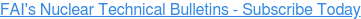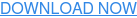### nuclear### chemical### Combustible dust### testing### news#### Recent Posts

By William E. Berger, Senior Vice President, Fauske & Associates, LLC

The dielectric constant is associated with specific materials such as those used in cable insulation, capacitors and printed circuit board substrates. It is a complex number with the imaginary part associated with dielectric losses and the real part (Ɛr) an indication of the degree to which a material can be polarized. Our focus will be on the real portion of the dielectric constant and what it means for cable applications.

Ɛr is a dimensionless number that is multiplied by the constant for free space Ɛ0 which is 8.854*10-12 F/m. The greater the degree of polarization, the greater the value of Ɛr . Before discussing polarization and its implication towards cable performance we will first briefly review the history of how the value of Ɛo was determined.In the early 1800’s, the fields of electromagnetic and electrostatic were developed based on experimental evidence. The two most fundamental experiments performed measured forces between two charges through the electric field created by the charges and the force on a wire carrying a current in the presence of a magnetic field. This led to the definition of the electric field E as the force per coulomb and the magnetic field B as the force per meter to the amps carried by the wire. The electric and magnetic forces identified in these experiments were only proportional to the sources creating these forces. To create an equivalence, proportionality constants were needed and this is where Ɛo and µo evolved. These two constants are tied together such that the definition of one determines the other. The relationship evolved from Maxwell’s equations which were used to derive the equation of a plane electromagnetic wave (See Chapter 11 of reference 1 for details). The equation for an electromagnetic wave includes a velocity term which for free space would be the speed of light. The equation for that velocity is:

Eq. 1When the ampere was defined the value of µo was established

Eq. 2Having this definition Ɛo then became, based on the above equation for the speed of light, 8.854 *10-12 F/m. A good source of information on the development of electrical units and from which some of this material was taken is the appendix to reference 2.

Our focus now turns to Ɛr . As mentioned Ɛr is a measure of the degree to which a material can be polarized which is the formation of electrical dipoles, referenced as shown in Figure 1.

Fig. 1These dipoles form when an electric field is applied E (volts/meter). Electronic polarization occurs in most all dielectrics materials.

Fig. 2For an atom the center of positive charge (nucleus) and that for the negative charge (electron cloud) coincide. With the application of an electric field the lighter negative center of charge separates slightly from the positive charge forming a dipole. Another mechanism for forming electric dipoles is ionic polarization. There are materials which have permanent dipoles. These are referred to as polar. The application of an electric field tends to cause these dipoles to rotate and align with the field, figure 3.

Fig. 3All these mechanisms contribute to the total polarization P which is the density of dipole moments. The magnitude of P is dependant on the applied electric field as well as the type of material which is reflected in the dielectric constant.

Eq. 3           P=Ɛo Ɛr

We can view a cable as a cylindrical capacitor with the insulation acting as the dielectric. As with all capacitors, there needs to be a charging current due to polarization. The higher the dielectric constant, the greater the charging current. In AC circuits, this current needs to be supplied every half cycle.

There is an emphasis on testing cables in the field to determine their acceptability. Methods using transmission line theory will also measure the cable capacitance. A variation in this value over time would indicate a change in the dielectric constant which implies changes in the insulating materials. Such changes could be the result of aging and the environment (i.e. temperature, radiation, etc. ). The dielectric constant is a also a function of frequency. As frequency increases, the value decreases due to polarization mechanisms no longer being able to follow the rapidly changing field. A shift in the frequency where these decreases occur can also be an indication of cable aging. These affects are being investigated through dielectric spectroscopy.

In summary, the dielectric constant indicates the degree of polarization and thus, the amount of charging current needed. Shifts in its value can serve as a metric for measuring cable health.References
1. Hayt, William “Engineering Electromagnetics”
2. Jackson, John “Classical Electrodynamics## Is My Dust Combustible?